Mathematical and Physical Journal
for High Schools
Issued by the MATFUND Foundation
 Already signed up? New to KöMaL?

# KöMaL Problems in Physics, January 2008

Show/hide problems of signs:## Problems with sign 'M'

Deadline expired on February 11, 2008.

M. 284. Make cylindrical lenses by filling bottles of different diameters with water. How does the position of the focus depend on the diameter of the bottle?

(6 pont)

statistics## Problems with sign 'P'

Deadline expired on February 11, 2008.

P. 4033. Three cells are connected in series. Their electromotive forces are 2 V, 10 V, and 5 V; their internal resistances are 0.2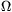, 0.3, and 0.5. Calculate the voltage across the third cell and graph this terminal voltage as a function of the external resistance connected to the circuit.

(4 pont)

statistics

P. 4034. A body of mass m=1.8 kg is placed to an inclined plane, the angle of inclination is=30o, and is attached to the top end of the slope wit a thread which is parallel to the slop. Then the slope is moved with a horizontal acceleration of a. Friction is negligible.

a) Find the acceleration if the body pushes the slope with a force of.

b) Find the tension.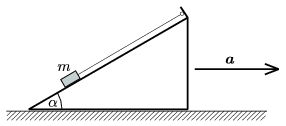(4 pont)

statistics

P. 4035. A ball, dropped from a height of 1.5 m, is bouncing for 3 seconds. At what rate should the ball be bounced in order to make it reach the height of 1.5 m?

(4 pont)

statistics

P. 4036. A small body of negligible size slides with a speed of v onto a long cart, which can move easily. The mass of the small body and the cart are the same. In the middle of the cart there is a slope which has a shape of a circular arc of central angle of 45o and of radius R. The small body slides along the slope and then it falls exactly to the end of the cart. What is the length of the cart? (Friction is negligible.)

Data: v=5 m/s, R=0.4 m.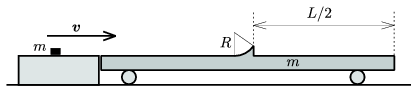(5 pont)

statistics

P. 4037. A cylindrical pot is slowly filled with water. The centre of mass of the empty pot is at a height of 10 cm, the mass of the pot is 1 kg, and its diameter is 20 cm. What is the height of the water in it, if the centre of mass of the system is at the lowest position? Where is the centre of mass at this case with respect to the level of water?

(5 pont)

statistics

P. 4038. In modern wastewater treatment plants water is thickened first to gain biomass which is rich in organic materials. With the help of bacteria, biogas is produced, and biogas is held in liquid sealed containers. (The container is similar to an upside-down glass which is placed to water.) If the amount of gas is increasing, then the container ascends, if the amount of gas is decreasing then it descends. The gas cannot escape because of the liquid at the bottom of the tank. For safety reasons the height of the liquid h must be at least 1 metre, and the top of the tank can be maximum H=6.5 metres above the the level of the water outside.

60% of the produced gas is metan, and 40% is carbon-dioxide. The volume of the empty cylinder shaped container is 2000 m3, the gauge pressure of the confined gas is 50 mbar, and the maximum temperature of the gas in a hot summer day can be 60 oC.

a) Find the mass of the moving part in tons.

b) How many cubic metre gas can be put to the container at a pressure of p=1.013 bar and at a temperature of 20 oC?

c) How much heat is released if the total amount of gas is burned?

The average external pressure is p0=1.013.105 Pa.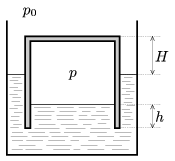(5 pont)

statistics

P. 4039. There is a sample of gas of mass 192 g, in a container of volume 80 l at a temperature of 402 oC and at a pressure of 4.2.105 Pa. The specific heat capacity of the gas at constant volume is 124.5 J/K.

a) What is the number of the degrees of freedom of the molecules?

b) What kind of gas can be in the container?

(4 pont)

statistics

P. 4040. Design a circuit using a battery of 6 V and several LED-s of different colours, whose forward voltages 2 V, 3 V, and 4 V voltages, and their current is 10 mA. The probability that resistor goes wrong in one year is 1% and the probability that a LED goes wrong in one year is 3%. What is the probability that the circuit operates without going wrong in the first year.

(4 pont)

statistics

P. 4041. A thin uniform rod of length 2and of mass m can move along a vertical circle of radius R without friction. Determine the frequency of the small amplitude oscillatory motion of the rod about the equilibrium position.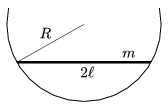(5 pont)

statistics

P. 4042. A filament lamp rated at 40 W transforms 2% of the total energy to visible light. Suppose that the time of the emission of one photon is 10-14 second. Find the average number of visible photons emitted by the filament at the same time.

(4 pont)

statistics